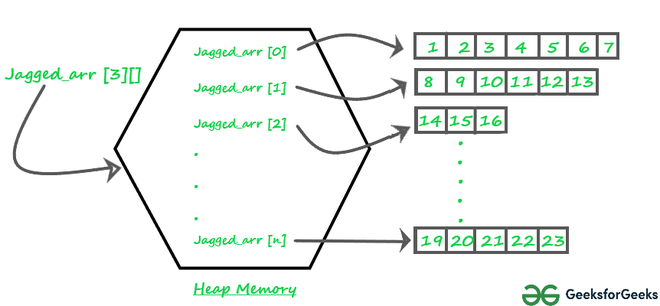Related Articles

# Jagged Array in Java

• Difficulty Level : Easy
• Last Updated : 05 Feb, 2021

Prerequisite: Arrays in Java

A jagged array is an array of arrays such that member arrays can be of different sizes, i.e., we can create a 2-D array but with a variable number of columns in each row. These types of arrays are also known as Jagged arrays.

Pictorial representation of Jagged array in Memory:Jagged_array

Declaration and Initialization of Jagged array :

```Syntax: data_type array_name[][] = new data_type[n][];  //n: no. of rows
array_name[] = new data_type[n1] //n1= no. of colmuns in row-1
array_name[] = new data_type[n2] //n2= no. of colmuns in row-2
array_name[] = new data_type[n3] //n3= no. of colmuns in row-3
.
.
.
array_name[] = new data_type[nk]  //nk=no. of colmuns in row-n```

Alternative, ways to Initialize a Jagged array :

```                    int arr_name[][] = new int[][]  {
new int[] {10, 20, 30 ,40},
new int[] {50, 60, 70, 80, 90, 100},
new int[] {110, 120}
};

OR

int[][] arr_name = {
new int[] {10, 20, 30 ,40},
new int[] {50, 60, 70, 80, 90, 100},
new int[] {110, 120}
};

OR

int[][] arr_name = {
{10, 20, 30 ,40},
{50, 60, 70, 80, 90, 100},
{110, 120}
};```

Following are Java programs to demonstrate the above concept.

## Java

 `// Program to demonstrate 2-D jagged array in Java``class` `Main {``    ``public` `static` `void` `main(String[] args)``    ``{``        ``// Declaring 2-D array with 2 rows``        ``int` `arr[][] = ``new` `int``[``2``][];` `        ``// Making the above array Jagged` `        ``// First row has 3 columns``        ``arr[``0``] = ``new` `int``[``3``];` `        ``// Second row has 2 columns``        ``arr[``1``] = ``new` `int``[``2``];` `        ``// Initializing array``        ``int` `count = ``0``;``        ``for` `(``int` `i = ``0``; i < arr.length; i++)``            ``for` `(``int` `j = ``0``; j < arr[i].length; j++)``                ``arr[i][j] = count++;` `        ``// Displaying the values of 2D Jagged array``        ``System.out.println(``"Contents of 2D Jagged Array"``);``        ``for` `(``int` `i = ``0``; i < arr.length; i++) {``            ``for` `(``int` `j = ``0``; j < arr[i].length; j++)``                ``System.out.print(arr[i][j] + ``" "``);``            ``System.out.println();``        ``}``    ``}``}`
Output
```Contents of 2D Jagged Array
0 1 2
3 4
```

Following is another example where i’th row has i columns, i.e., the first row has 1 element, the second row has two elements and so on.

## Java

 `// Another Java program to demonstrate 2-D jagged``// array such that first row has 1 element, second``// row has two elements and so on.``class` `Main {``    ``public` `static` `void` `main(String[] args)``    ``{``        ``int` `r = ``5``;` `        ``// Declaring 2-D array with 5 rows``        ``int` `arr[][] = ``new` `int``[r][];` `        ``// Creating a 2D array such that first row``        ``// has 1 element, second row has two``        ``// elements and so on.``        ``for` `(``int` `i = ``0``; i < arr.length; i++)``            ``arr[i] = ``new` `int``[i + ``1``];` `        ``// Initializing array``        ``int` `count = ``0``;``        ``for` `(``int` `i = ``0``; i < arr.length; i++)``            ``for` `(``int` `j = ``0``; j < arr[i].length; j++)``                ``arr[i][j] = count++;` `        ``// Displaying the values of 2D Jagged array``        ``System.out.println(``"Contents of 2D Jagged Array"``);``        ``for` `(``int` `i = ``0``; i < arr.length; i++) {``            ``for` `(``int` `j = ``0``; j < arr[i].length; j++)``                ``System.out.print(arr[i][j] + ``" "``);``            ``System.out.println();``        ``}``    ``}``}`
Output
```Contents of 2D Jagged Array
0
1 2
3 4 5
6 7 8 9
10 11 12 13 14
```

This article is contributed by Rahul Agrawal. If you like GeeksforGeeks and would like to contribute, you can also write an article and mail your article to contribute@geeksforgeeks.org. See your article appearing on the GeeksforGeeks main page and help other Geeks.